# I need the answer to problem 4 (exercises 1, 2, 3) Clear and step by step...

I need the answer to problem 4 (exercises 1, 2, 3)

Clear and step by step please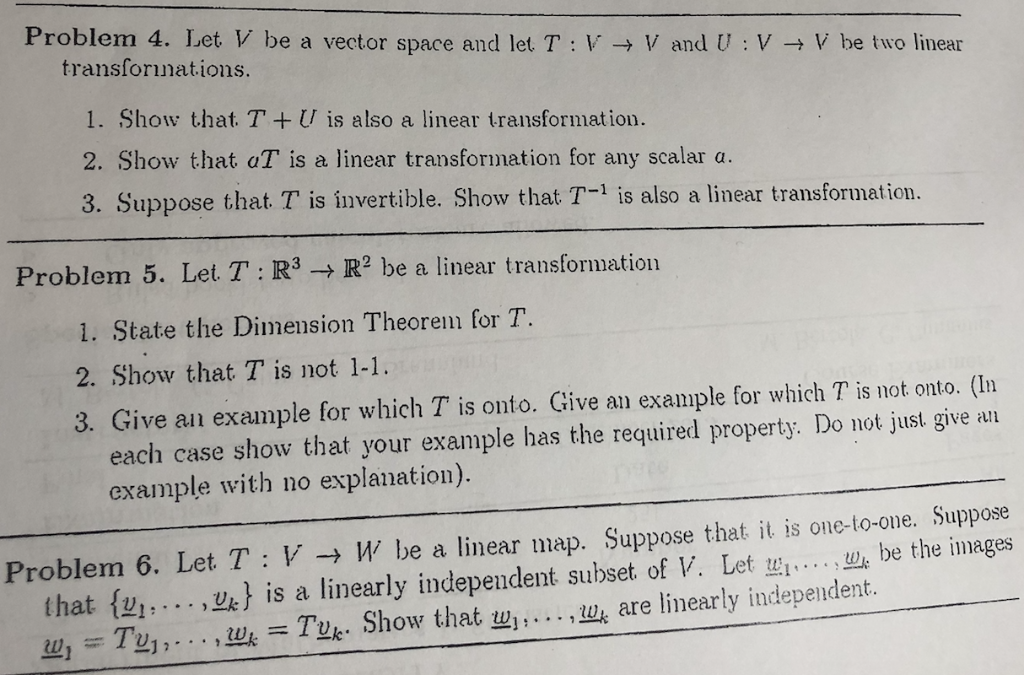Problem 4. Let V be a vector space and let T : V → V and U : V → V be two linear transforinations 1. Show that. TU is also a linear transformation. 2. Show that aT is a linear transformation for any scalar a. 3. Suppose that T is invertible. Show that T-1 is also a linear transformation. Problem 5. Let T : R3 → R2 be a linear transformatio! 1. State the Dimension Theorem for T. 2. Show that T is not 1-1. 3. Give an example for which T is onto. Give an example for which T is not onto. (In each case show that your example has the required property. Do not just give an example with no explanation). Problem 6, Let T : V → W be a linear map. Suppose that it is one-to-one. Suppose th be the images . . . . ,蚴is a linearly independent subset of V. Let wi 2], . . . ,we-Tuk. Show that w].. that { uh are linearly independent.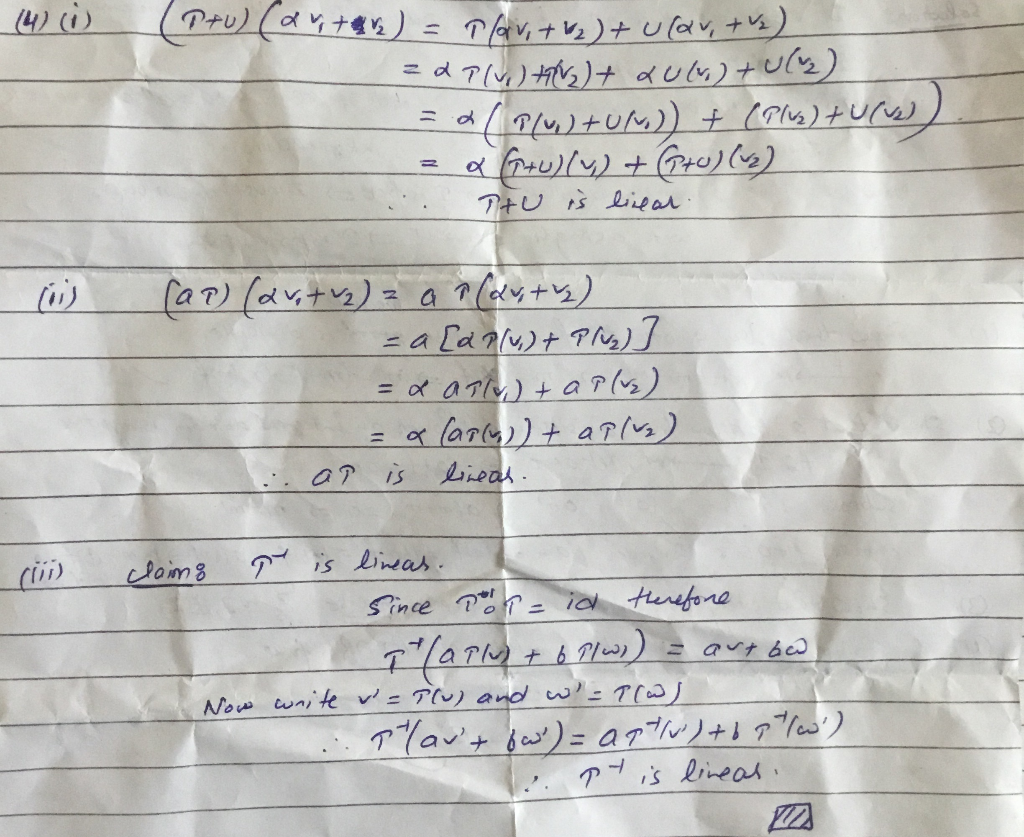##### Add Answer of: I need the answer to problem 4 (exercises 1, 2, 3) Clear and step by step...
Similar Homework Help Questions
• ### Problem 4. Let V be a vector space and let T : V → V and U : V → V be two linear transforinations...I need the answer to problem 6 Clear and step by step please Problem 4. Let V be a vector space and let T : V → V and U : V → V be two linear transforinations 1. Show that. TU is also a linear transformation. 2. Show that aT is a linear transformation for any scalar a. 3. Suppose that T is invertible. Show that T-1 is also a linear transformation. Problem 5. Let T : R3 →...

• ### Please give answer with the details. Thanks a lot! Let T: V-W be a linear transformation between vector spaces V and W...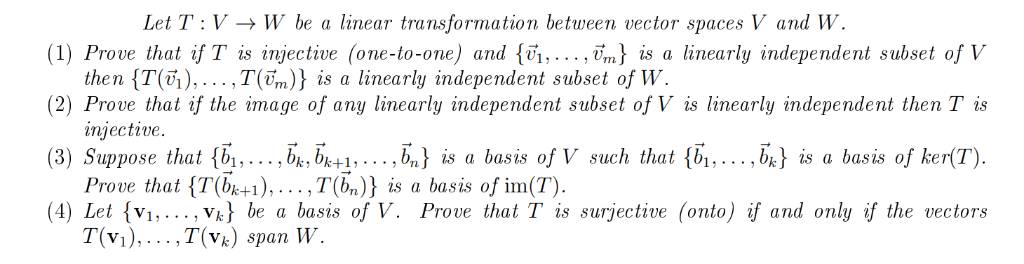Please give answer with the details. Thanks a lot! Let T: V-W be a linear transformation between vector spaces V and W (1) Prove that if T is injective (one-to-one) and {vi,.. ., vm) is a linearly independent subset of V the n {T(6),…,T(ền)} is a linearly independent subset of W (2) Prove that if the image of any linearly independent subset of V is linearly independent then Tis injective. (3) Suppose that {b1,... bkbk+1,. . . ,b,) is a...

• ### 1. Determine whether the following set is linearly independent or not. Prove your clas a. [1+1,...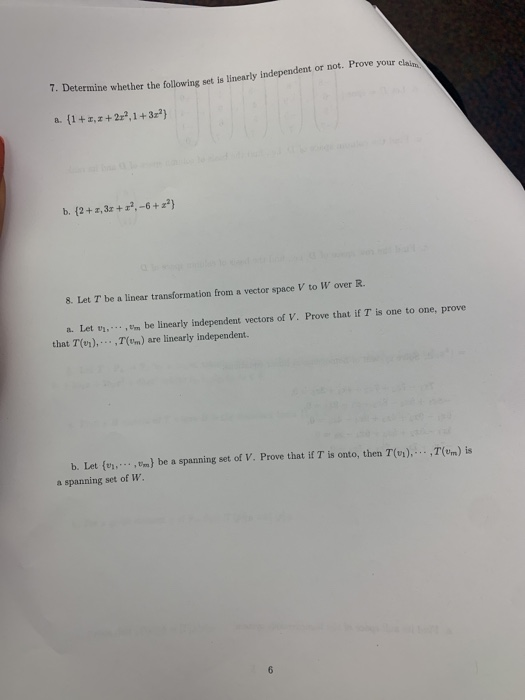1. Determine whether the following set is linearly independent or not. Prove your clas a. [1+1, 2+2-2,1 +32"} b. {2+1, 3x +3',-6 +2"} 8. Let T be a linear transformation from a vector space V to W over R. . Let .. . be linearly independent vectors of V. Prove that if T is one to one, prove that (un)....(...) are linearly independent. (m) is ) be a spanning set of V. Prove that it is onto, then Tu... h...

• ### Problem 1: Let W = {p(t) € Pz : p'le) = 0}. We know from Problem...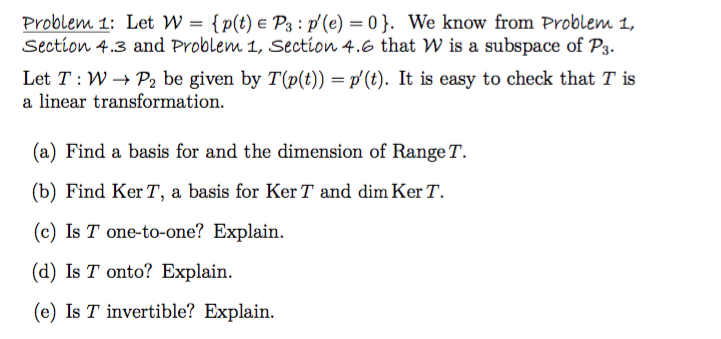Problem 1: Let W = {p(t) € Pz : p'le) = 0}. We know from Problem 1, Section 4.3 and Problem 1, Section 4.6 that W is a subspace of P3. Let T:W+Pbe given by T(p(t)) = p' (t). It is easy to check that T is a linear transformation. (a) Find a basis for and the dimension of Range T. (b) Find Ker T, a basis for Ker T and dim KerT. (c) Is T one-to-one? Explain. (d) Is...

• ### transformation

2. Let V and W be vector spaces and T: V－>W be a linear transformation. For a given U subset of V,we denote byT(U)the subset of W deined byT(U)= {T(u)|uε U}.(a) Prove that T is one-to-one if and only if for every linearly independent subset U of V, the subsetT(U) of W is linearly independent.(b) Assume that V={v1,...,vn} is a basis for V and that T is one-to-one and onto. Prove that T(V) is a basis for W.c) Assume that...

• ### Problem 1. The figure below shows the vectors u, v, and w, along with the images...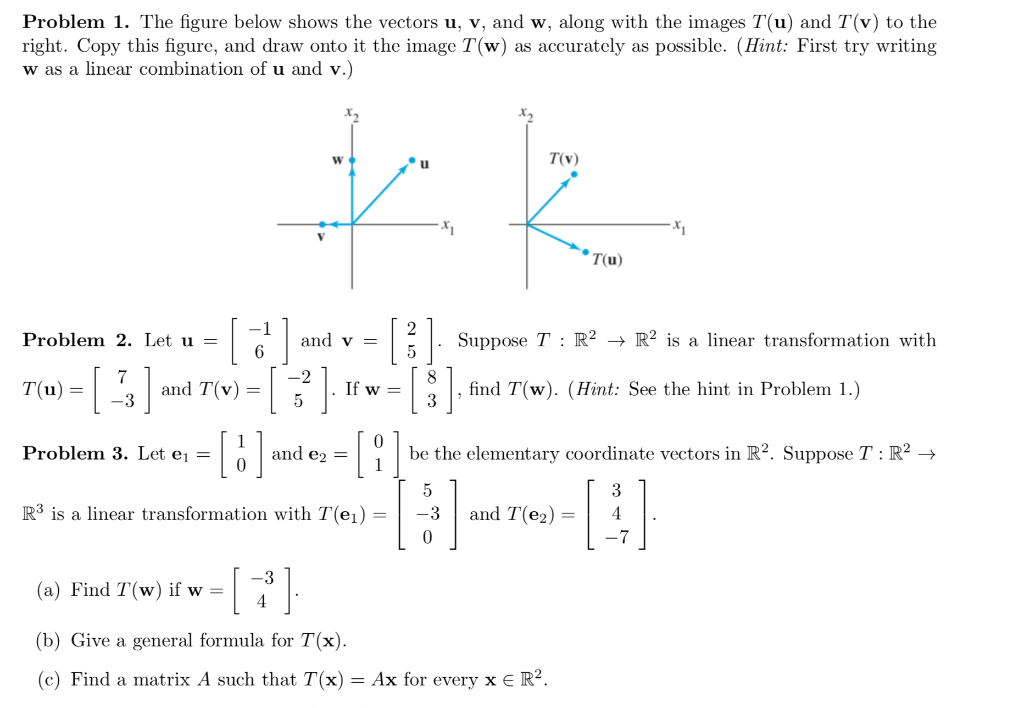Problem 1. The figure below shows the vectors u, v, and w, along with the images T(u) and T(v) to the right. Copy this figure, and draw onto it the image T(w) as accurately as possible. (Hint: First try writing w as a linear combination of u and v.) TV (u) Problem 2. Let u = | and v Suppose T : R2 + R2 is a linear transformation with 6 1 3) Tu = T(u) = -3 and T(v)...

• ### Please answer me fully with the details. Thanks! True of False? Justify yo ur answer. —D т. If {ii, .., in} is a linear...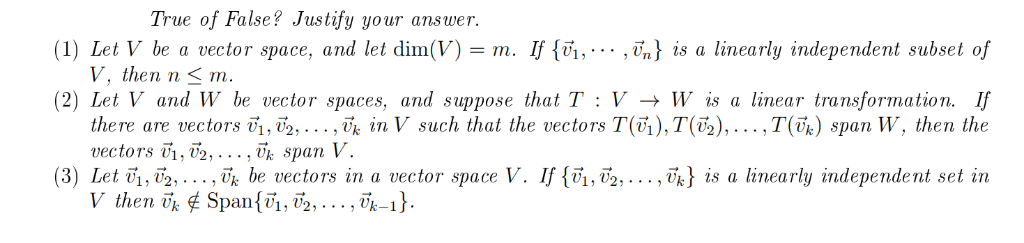Please answer me fully with the details. Thanks! True of False? Justify yo ur answer. —D т. If {ii, .., in} is a linearly independent subset of (1) Let V bea vector spacе, аnd let dim(V) V. then n < т. (2) Let V and W be vector spaces, and suppose that T : V -+ W is a linear transformation. If there are vectors i, 2, ..., Tj in V such that the vectors T(),T(T2),...,T(vj) span W, then the...

• ### 101-2019-3-b (1).pdf-Adobe Acrobat Reader DC Eile Edit iew Window Help Home Tools 101-2019-3-b (1...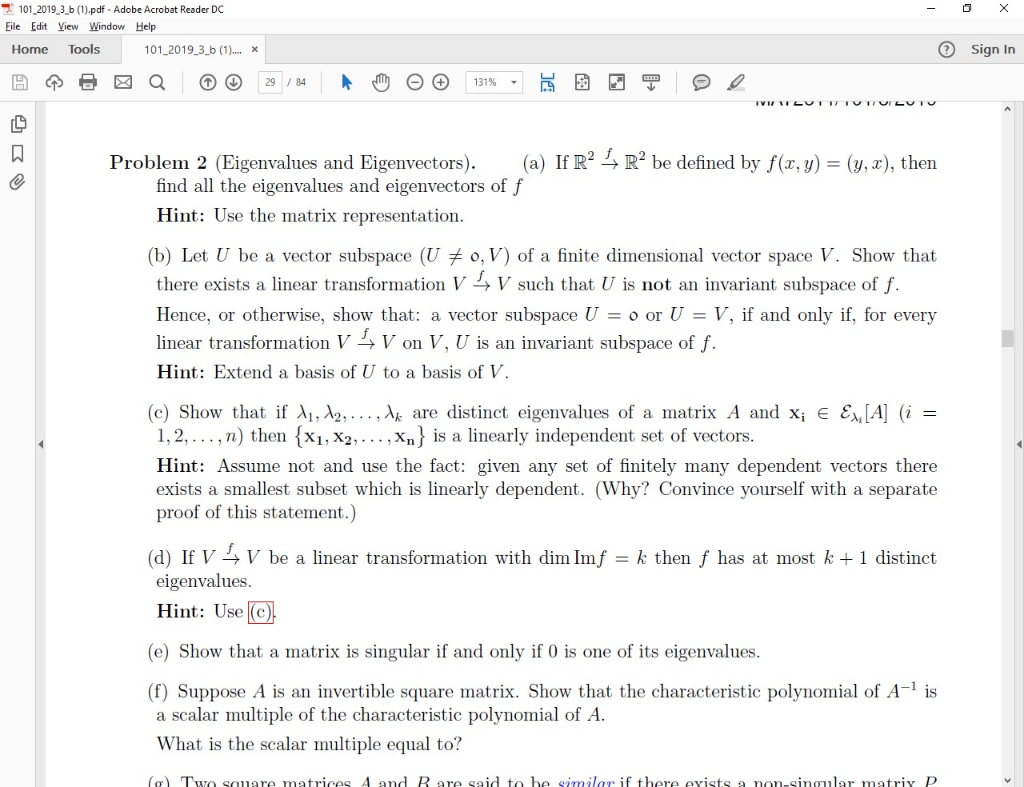101-2019-3-b (1).pdf-Adobe Acrobat Reader DC Eile Edit iew Window Help Home Tools 101-2019-3-b (1) Sign In x Problem 2 (Eigenvalues and Eigenvectors). (a) If R2 4 R2 be defined by f(x,y) (y, x), then find all the eigenvalues and eigenvectors of f Hint: Use the matrix representation (b) Let U be a vector subspace (U o, V) of a finite dimensional vector space V. Show that there exists a linear transformation V -> V such that U is not an...

• ### Proble m 3. Let T: V ->W be (1) Prove that if T is then T(),... ,T(Fm)} is a linearly indepen dent subset of W (2) P...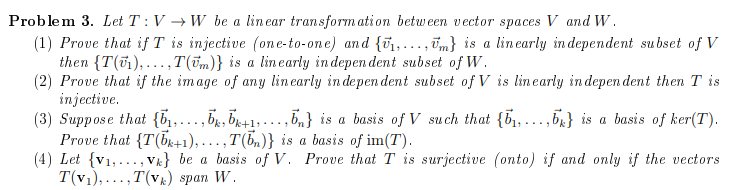Proble m 3. Let T: V ->W be (1) Prove that if T is then T(),... ,T(Fm)} is a linearly indepen dent subset of W (2) Prove that if the image of any linearly in depen dent subset of V is linearly indepen dent then T is injective (3) Suppose that {,... ,b,b^1,...,5} is Prove that T(b1), .. . , T(b,)} is a basis of im(T) (4) Let v1,. Vk} be T(v1),..,T(vk) span W lin ear transform ation between vector...

• ### 7. Let T:V : - W be a linear transformation, and let vi, U2,..., Un be...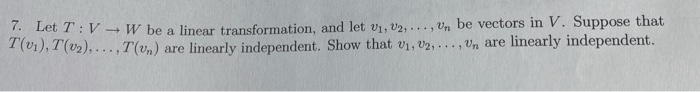7. Let T:V : - W be a linear transformation, and let vi, U2,..., Un be vectors in V. Suppose that T(01), T (v2),..., 1 (un) are linearly independent. Show that 01, V2, ..., Un are linearly independent.

Free Homework App